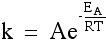Posted on

# Using Simultaneous Equations and the Arrhenius EquationThe relationship between the activation energy for a reaction, Ea, temperature and the rate constant is shown by the Arrhenius equation:(A derivation of this equation pops up in ACER Practice Test 1, Questions 49-51)

The Arrhenius Equation looks pretty nasty…after all it has a FRACTION raised to the power…with base “e”…eeeee indeed!

Also, dragons:

But we must remember that the rules for manipulation of NATURAL LOGS (ln) are the SAME as LOGS to the BASE 10.

If you need to refresh these rules, please see my previous post “Logs in less than 5 minutes” which has a great video explanation of the rules. The resources section of the cbsquared Chemistry for GAMSAT online course also contains some practice drills for using logs, and also manipulating scientific notation which may be helpful.)

Ok. Now that we have that sorted, we might have the activation energy levels high enough to launch into the next video (don’t worry it’s only a little more than THREE MINUTES!) which demonstrates how we can use simultaneous equations to use the relationship between temperature and rate constant to solve for Ea, Activation Energy.

For a more indepth discussion of the Arrhenius equation and the forms it takes, the Khan Academy also has a really great resource (this link also includes some other kinetics concepts such as rate laws and reaction mechanisms).

Also, for a detailed explanation of the pre-exponential factor, A, check out this chemwiki resource.

by